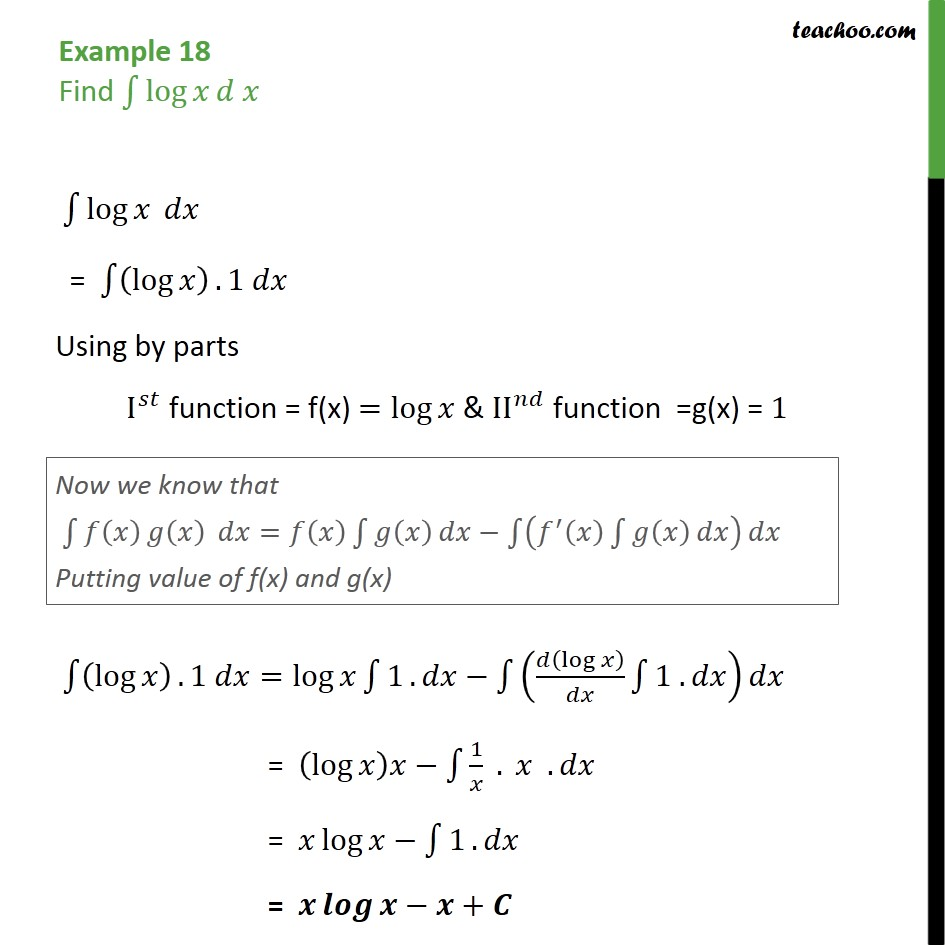Examples

Chapter 7 Class 12 Integrals
Serial order wise

## For Finding Integration of lnx (log x),

we use Integration by Parts

1. Write ∫ log x dx = ∫ (log x) . 1 dx
2. Take first function as log x, second function as 1.
3. Use integration by Parts and solveThere are other formulas which are used to find Integral, refer Integral Table .

Learn in your speed, with individual attention - Teachoo Maths 1-on-1 Class

### Transcript

Example 18 Find Intgeral of log x ln x log = log .1 Using by parts I function = f(x) = log & II function =g(x) = 1 log .1 = log 1 . log 1 . = log 1 . . = log 1 . = +